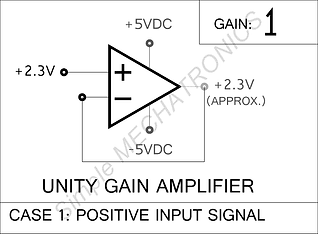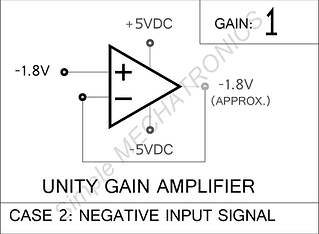top of page

## op-amp:

OP-AMP (Operational Amplifier) is basically a differentiator, which reads two electrical signals as inputs and sends the output signal accordingly with amplification.OP-AMP should have at least 5 pins. i.e.,

1. Non-Inverting input pin

2. Inverting Input pin

3. Output pin

4. Positive power supply pin

5. Negative (or sometimes ground ) pinThe two inputs are marked as NON-INVERTING INPUT or PLUS (+)  and  INVERTING INPUT or MINUS (-).   The output swings towards Positive, if the signal voltage at NON-INVERTING INPUT is higher than at INVERTING INPUT and vice-versa.

## GAIN:

Some part of output signal called as feedback is used as input signal to INVERTING or NON-INVERTING inputs to adjust the amplification factor, which is also called as gain.  The range of gain varies from 1 (also called as unity gain) to infinity depending on the feedback set to the input pins.So, the value of the output will be depending on the difference in voltage at INVERTING and NON-INVERTING inputs and amplification factor (gain) set by the RLC (resistor, inductor, capacitor) circuit used between output pin to INVERTING or NON-INVERTING input pin(s) as feedback.

## OPEN LOOP GAIN AMPLIFIER:If the output pin does not send any feedback to any input pin, then the amplification is infinity.  It is also known as saturation stage.

Means, a small difference in signal at INVERTING and NON-INVERTING inputs, swings the output to either Positive or Negative (ground).

In this condition OP-AMP works as BISTABLE (either ON or OFF) amplifier, which may be considered as digital switch.

The four conditions of open loop amplifier is presented here.## UNITY GAIN AMPLIFIER:

Similarly, if the output signal is directly connected to NON-INVERTING input of the OP-AMP, the exact difference in the input voltage is available at output.  This is called UNITY GAIN amplifier.

This is also called Unity Gain BUFFER, since, the output voltage matches the input voltage and the output current is amplified to drive relays and other electronic equipment with (small current) input signal.

So, the main advantage and usage of Unity Gain Amplifier is, high input impedance and least output impedance. Which means, a very weak signal (with low current) will be amplified to high current output to drive other devices, where output voltage being the difference of the voltages at both the input pins.## NEGATIVE FEED-BACK GAIN AMPLIFIER:

A part of output signal is sent to one of the inputs, which is called as feedback.

Most of the times the feedback is sent to INVERTING input, which is called as NEGATIVE FEED-BACK AMPLIFIER.

There are two cases of Negative Feed-back amplifiers.  In case, the input signal is connected to NON-INVERTING input, the output signal will be amplified (gain) and always more than one with maximum input impedance.

The Negative feed-back amplifier configuration is highly used in electronics circuits to control machinery and read & amplify weak signals.## POSITIVE FEED-BACK GAIN AMPLIFIER:

In case, the input signal is connected to INVERTING input through the resistance, the output signal will be inverted and amplification factor (gain) depends on the resistance network (R1 and R2).

Similarly, the feedback may be connected to NON-INVERTING input.  The output signal is inverted and swings to extremes with small delay, which is called HYSTERESIS.  This is called as POSITIVE FEED-BACK AMPLIFIER.

The Positive Feed-back Amplifiers are used as Latches, Oscillating circuits, Filters etc.bottom of page